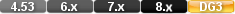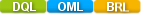Easy to Create, Easy to Change - Easy to use!## Acos (arccosine)acos(field) acos(numeric value) acos(-1) acos(0.5) acos(myvalue+yourvalue*theirvalue)

The acos function calculates the arccosine of a numeric value. The result is an angle expressed in radians between 0 and p.

# Parameters

Numeric Value between -1 via 0 to +1

Numeric value.

# Examples

Example 1

acos(0.5)

Result: 1.047195755

Example 2

acos(-0.8)

Result: 2.49809154

# acos (arccosine)

#### Type

Trigonometric Function

Purpose

The acos function calculates the arccosine of a numeric value. The result is an angle expressed in radians between 0 and p.

Syntax

acos( NUMERIC VALUE)

Returns

A numeric value.

Usage

The numeric value in a Trigonometric function can be a constant value (as shown below), a variable, a field value or an expression.

The valid range of the input value is -1 to 1

Examples

acos( 0.5)

Returns: 1.047195755

acos( -0.8)

Returns: 2.49809154